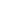Flat 50% off

Ends in

• NEET
•• 11th
•• Physics
• Back to Doubt Clearing

## plz solve this problemThe acceleration due to gravity g' at height h from the surface of radius R is given by $g' = \frac {gR^2}{(R + h)^2}$ where g is the acceleration due to gravity on the earth's surface. Find out the value of g' using the binominal theorem, if \(h<

### Asked By y mainu

Updated Wed, 14 Nov 2018 12:46 pm